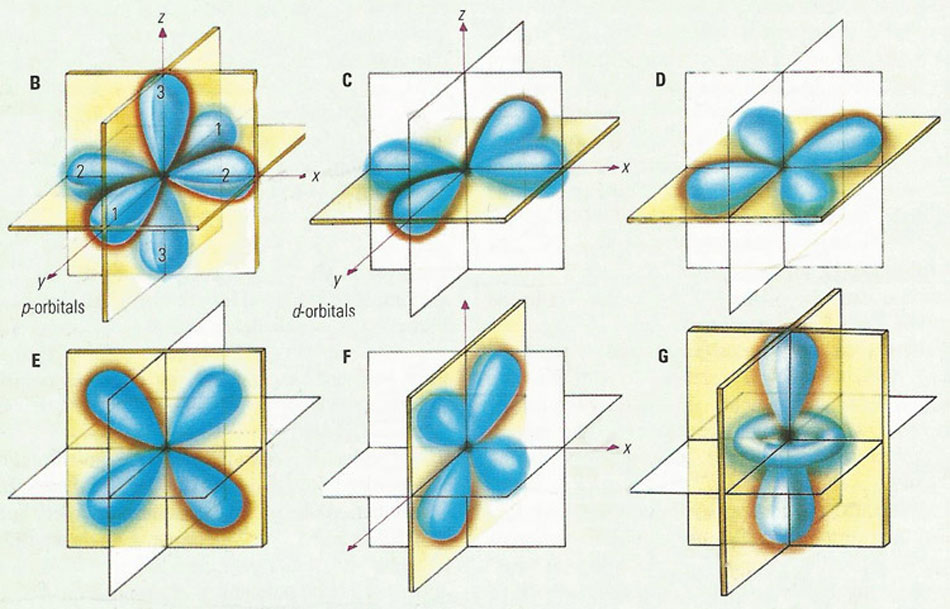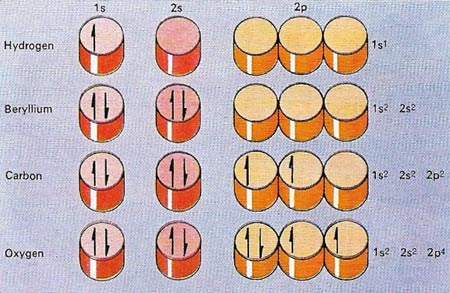A# orbitalFig 1. p- and d-orbitals.Fig 2. The simplest types of orbital: the s-orbital.Fig 3. The positions of electrons in a neural atom of any element can be shown in a 'pigeon-hole' notation. The orbitals are signified by a number – the principal quantum number that indicates the average distance of the electron from the nucleus – and a letter that indicates the shape of the orbital. The letters used for identification are derived from the initial letters of the four types of spectral lines, s, p, d and f and then proceed alphabetically. Because electrons – shown by half arrows – can spin in opposite directions, each pigeon-hole can hold two electrons.Fig 4. Atoms have more and more electrons as they build up to form the heavier elements and the order in which they occupy orbitals depends on the particular binding energy. In general, the closer an electron is to the nucleus, the greater is the energy. As electrons get farther away from the nucleus, the energy relationships become more complex. So that, for example, the 4s orbital is more strongly binding than the 3d orbital, and is filled with electrons before it. The chart shows the general order for the filling of orbitals as atoms get larger. However, even this is an approximation and there are a few exceptions to it.

An orbital is a wavefunction that describes what an electron with a given energy is doing, and where it is most likely to be found, inside an atom or molecule. Equivalently, an orbital can be thought of as one of the regions in the space around an atomic nucleus in which electrons are allowed.

In 1925, the Austrian physicist Wolfgang Pauli formulated rules for electron orbitals. His major rule was that no two electrons in the same atom can be in exactly the same quantum state. The quantum state of an atom is defined by four quantum numbers. The first of these, known as the principal quantum number, describes the average distance between the electron and the nucleus. The second number is related to the shape of the orbital, which is not always spherical, and the third determines its orientation. The final quantum number is the spin of the electron, which can only be +½ or -½. The possible values for the second and third quantum numbers depend on the value of the principal quantum number in such a way that, when it is 1, there are only two possible quantum states (and orbitals); when it is 2, there are 8; when it is 3, there are 18; and so on, according to the formula 2n2, where n is the principal quantum number.

The energy of any electron depends upon the first two quantum numbers and, because its behaviour depends largely on its energy, chemicals have developed a kind of shorthand for describing an electron's energy level. Each electron in an atom can be described by a number followed by a letter. The number is the same as the principal quantum number, but the values 0, 1, 2 and 3 for the second quantum number are represented (for historical reasons) by the letters s, p, and 7. By knowing the numbers of electrons in different orbitals, a chemist can predict the behaviour of any particular atom.

The simplest type of orbital – the s-orbital – is spherical and can be occupied by a maximum of two electrons. p- and d-orbitals are more complex in shape. There are three p-orbitals in each energy level (above the first) set at right-anges to each other. d-orbitals come in sets of five (C through G in Fig 1).

In molecules, the bonding electrons move in the combined electric field of all the nuclei. The atomic orbitals then become molecular orbitals – regions encompassing two nuclei, having a characteristic energy and containing two electrons. These molecular orbitals, which can be thought of as formed by the overlap of atomic orbitals, constitute chemical bonds.